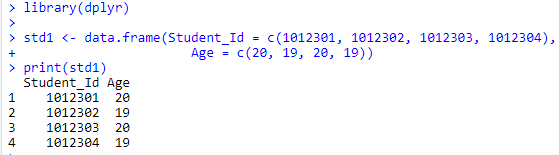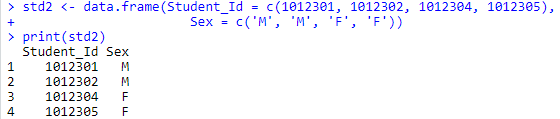top of pageSearch

# Merging Data Using DPLYR

In this blog, you will learn how to Merge Data using the dplyr R package. When you will be working with multiple datasets then often, you will need to combine these datasets to perform the analysis that you are interested in. The dplyr package provides several join functions to combine relational data. The fundamental syntax for each of these function is:

`xxxx_join(x = , y = , by = )`

The DPLYR Join:

The dplyr join functions can be broken down into two categories, mutating joins and filtering joins:

1] Mutating Joins :- Add new variables to one data frame from matching observations in another.

• inner_join : Return only rows that appear in both datasets.

• full_join : Returns all rows in both data sets

• left_join : Returns all rows from x and matching rows from y

• right_join : Returns all rows from y and the matching rows in x

2] Filtering Joins :- Filter observations from one data frame based on whether or not they match an observation in another.

• semi_join : Returns rows of x that are in y

• anti_join : Returns rows of x that don’t match in y

# Required R package

First, you need to install the dplyr package and load the dplyr library then after you can able to perform the following data merging operations.

Mutating Inner Joins

An inner join matches the pairs of observations whenever their keys are equal. As an example, let’s look at the following two data frames.

```install.packages('dplyr')
library(dplyr)std1 <- data.frame(Student_Id = c(1012301, 1012302, 1012303, 1012304),
Age = c(20, 19, 20, 19))
print(std1)```

Output:```std2 <- data.frame(Student_Id = c(1012301, 1012302, 1012304, 1012305),
Sex = c('M', 'M', 'F', 'F'))
print(std2)```

Output:In this example, Student_Id 1012301, 1012302 and 1012304 are common among both datasets.

```InnerJoin <- inner_join(x = std1, y = std2, by = "Student_Id")
print(InnerJoin)```

Output:Mutating Outer Joins(Full, Left, Right)

An outer join keeps observations that appear in at least one of the datasets. Let’s again look at the same two data frames.

Full Join:- A full join results in Student_Id 1012303 and 1012305 appearing in the output dataset despite being unique to std1 and std2 respectively:

```FullJoin <- full_join(x = std1, y = std2, by = "Student_Id")
print(FullJoin)```

Output:Left Join:- Left join keeps all observations in x, and matching observations from y. A left join results in a dataset with Student_Id’s 1012301, 1012302, 1012303 and 1012304, plus the data from std2 for Student_Id’s 1012301, 1012302 and 1012304.

```LeftJoin <- left_join(x = std1, y = std2, by = "Student_Id")
print(LeftJoin)```

Output:Right Join:- Right join keeps all observations in y, and matching observations from x. A left join results in a dataset with Student_Id’s 1012301, 1012302, 1012304 and 1012305, plus the data from std2 for Student_Id’s 1012301, 1012302 and 1012304.

```RightJoin <- right_join(x = std1, y = std2, by = "Student_Id")
print(RightJoin)```

Output:# Filtering Joins

Filtering joins match observations in the same way as mutating joins, but only affect the observations(not the variables):

```SemiJoin <- semi_join(x = std1, y = std2, by = "Student_Id")
print(SemiJoin)```

Output:```AntiJoin <- anti_join(x = std1, y = std2, by = "Student_Id")
print(AntiJoin)```

Output:# Note:

1. Pairs of datasets can be joined by one, or multiple key variables.

2. When no key is specified, the default(by=NULL) uses all variables that appear in both datasets.

3. If you wish to merge on multiple key variables, you can specify this using the combine function:

`xxxx_join(x = , y = , by = c('a', 'b'))`

4. Duplicate keys: When you join duplicated keys, you get all possible combinations, the Cartesian product.

The previous part of the series part1 and part2 covered the data manipulation using the dplyr and tidyr package.

If you like the blog or found it helpful please leave a clap!

Thank you